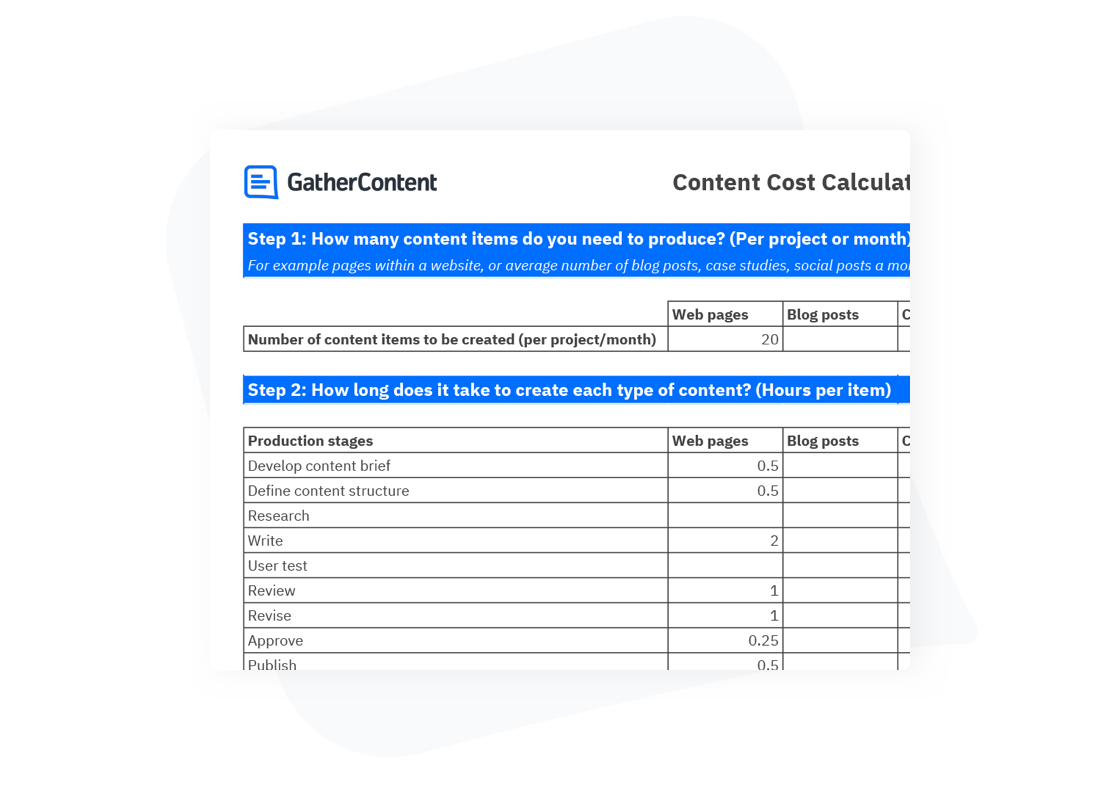# Content Cost Calculator

## A template to calculate the time and cost of creating content in your organisation.## How the template can help

Use our Content Cost Calculator template to:

• Calculate how many content items you need to produce (per project/month)
• Calculate how long it takes to create each piece of content from brief through to publishing
• Calculate the financial cost of hours required
• Estimate time and cost savings by implementing GatherContent for your content operations